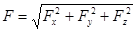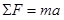# 01. Concepts and Principles

$$\newcommand{\vecs}{\overset { \rightharpoonup} {\mathbf{#1}} }$$ $$\newcommand{\vecd}{\overset{-\!-\!\rightharpoonup}{\vphantom{a}\smash {#1}}}$$$$\newcommand{\id}{\mathrm{id}}$$ $$\newcommand{\Span}{\mathrm{span}}$$ $$\newcommand{\kernel}{\mathrm{null}\,}$$ $$\newcommand{\range}{\mathrm{range}\,}$$ $$\newcommand{\RealPart}{\mathrm{Re}}$$ $$\newcommand{\ImaginaryPart}{\mathrm{Im}}$$ $$\newcommand{\Argument}{\mathrm{Arg}}$$ $$\newcommand{\norm}{\| #1 \|}$$ $$\newcommand{\inner}{\langle #1, #2 \rangle}$$ $$\newcommand{\Span}{\mathrm{span}}$$ $$\newcommand{\id}{\mathrm{id}}$$ $$\newcommand{\Span}{\mathrm{span}}$$ $$\newcommand{\kernel}{\mathrm{null}\,}$$ $$\newcommand{\range}{\mathrm{range}\,}$$ $$\newcommand{\RealPart}{\mathrm{Re}}$$ $$\newcommand{\ImaginaryPart}{\mathrm{Im}}$$ $$\newcommand{\Argument}{\mathrm{Arg}}$$ $$\newcommand{\norm}{\| #1 \|}$$ $$\newcommand{\inner}{\langle #1, #2 \rangle}$$ $$\newcommand{\Span}{\mathrm{span}}$$$$\newcommand{\AA}{\unicode[.8,0]{x212B}}$$

# Concepts and Principles

Just like in kinematics, it’s an empirical fact about nature that when a force acts on an object in one direction (for example, the horizontal) this action does not appear to cause changes in the motion in a perpendicular direction (the vertical). Therefore, to investigate the effects of forces on the motion of an object in the vertical direction, you can ignore all forces acting in the horizontal direction. Of course, many forces will simultaneously act in both the horizontal and vertical directions. As in kinematics, the effect of these forces can be examined by concentrating on the components of the forces in the various directions. Again, as long as the directions of interest are perpendicular, the force components can be determined through right-angle trigonometry, and the magnitude of the force can always be determined by:Thus, Newton’s second law,is independently valid in any member of a set of perpendicular directions. The total force in the horizontal direction, for example, is equal to the mass times the acceleration in that direction. Note that the mass has been verified to be independent of direction, meaning that objects possess the same inertia in all directions.

This page titled 01. Concepts and Principles is shared under a CC BY-NC-SA license and was authored, remixed, and/or curated by Paul D'Alessandris.